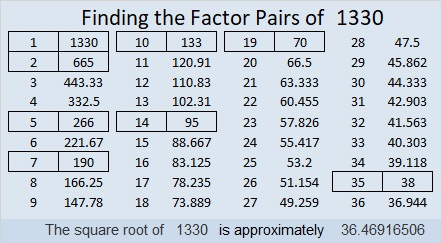# 1330 is the 19th tetrahedral Number

The product of any three consecutive counting numbers is always divisible by 6. Why? Because one of the numbers has to be divisible by 3 and at least one number has to be divisible by 2. Dividing the product by 6 always results in a  tetrahedral number. 1330 is a good example:
(19)(20)(21)/6 = 1330

Since the first number in that product was 19, we know that 1330 is the 19th tetrahedral number, and it is the sum of the first 19 triangular numbers:You can count all 1330 little green squares if you want in the graphic above if you choose.

Here are some more facts about the number 1330:

• 1330 is a composite number.
• Prime factorization: 1330 = 2 × 5 × 7 × 19
• The exponents in the prime factorization are 1, 1, 1, and 1. Adding one to each and multiplying we get (1 + 1)(1 + 1)(1 + 1)(1 + 1) = 2 × 2 × 2 × 2 = 16. Therefore 1330 has exactly 16 factors.
• Factors of 1330: 1, 2, 5, 7, 10, 14, 19, 35, 38, 70, 95, 133, 190, 266, 665, 1330
• Factor pairs: 1330 = 1 × 1330, 2 × 665, 5 × 266, 7 × 190, 10 × 133, 14 × 95, 19 × 70, or 35 × 38
• 1330 has no square factors that allow its square root to be simplified. √1330 ≈ 36.469171330 is the sum of the twenty-two prime numbers from 17 to 107. How cool is that?

1330 is the hypotenuse of a Pythagorean triple:
798-1064-1330 which is (3-4-5) times 266